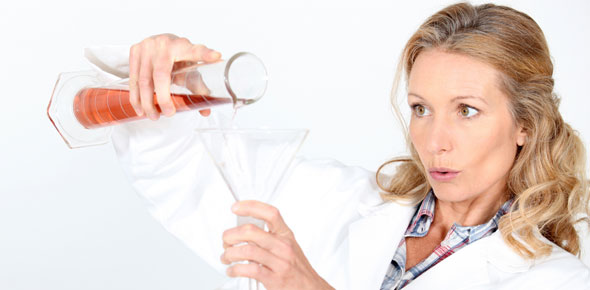# Chemical Reactions

24 Questions | Total Attempts: 627SettingsReview Quiz for Chemical Reactions Chapter Test

• 1.
Which of the following is a word equation?
• A.

Sodium oxide → sodium + oxygen

• B.

Sodium oxide decomposes to form sodium and oxygen

• C.

Na2O → Na + O2

• D.

2Na2O → 4Na + O2

• 2.
In an exothermic reaction, heat is
• A.

Absorbed

• B.

Released

• C.

Destroyed

• D.

Conserved

• 3.
The breaking down of a compound into simpler substances is
• A.

Displacement

• B.

Synthesis

• C.

Decomposition

• D.

Endothermic

• 4.
Each of the substances to the left of the arrow in a chemical equation is a
• A.

Catalyst

• B.

Product

• C.

Reactant

• D.

Coefficient

• 5.
Numbers that precede (come before) symbols and formulas in a chemical equation are
• A.

Subscripts

• B.

Superscripts

• C.

Coefficients

• D.

Fractions

• 6.
Which of the following types of reactions results in a single product?
• A.

A synthesis reaction

• B.

A decomposition reaction

• C.

A combustion reaction

• D.

A single replacement reaction

• E.

A double replacement reaction

• 7.
What does → signify?
• A.

"and"

• B.

"yields/produces"

• C.

Catalyst

• D.

• 8.
What type of reaction is the following?CH4 + 2O2 → CO2 + 2H2O
• A.

Synthesis

• B.

Decomposition

• C.

Single replacement

• D.

Double replacement

• E.

Combustion

• 9.
What type of reaction is the following?Cl2 + 2NaBr → 2NaCl + Br2
• A.

Synthesis

• B.

Decomposition

• C.

Single-replacement

• D.

Double-replacement

• E.

Combustion

• 10.
• A.

Synthesis

• B.

Decomposition

• C.

Single-replacement

• D.

Double-replacement

• E.

Combustion

• 11.
What coefficients would be added to balance the following equation?Fe + O2 → Fe2O3
• A.

2, 3, 1

• B.

4, 3, 2

• C.

• D.

You wouldn't add a coefficient you would just change the subscripts

• 12.
What coefficients would balance the following equation?Sr + H2O → Sr(OH)2 + H2
• A.

1, 4, 2, 2

• B.

2, 4, 2, 4

• C.

1, 2, 1, 1

• D.

• 13.
Which of the following is NOT an observable clue that a chemical reaction has occurred?
• A.

Release of a gas

• B.

Odor change

• C.

Precipitate formation

• D.

Mixing two reactants

• 14.
An insoluble substance formed from two solutions is a
• A.

Reactant

• B.

Precipitate

• C.

Compound

• D.

Product

• 15.
In a double-replacement reaction
• A.

The reactants are usually one metal and one nonmetal

• B.

One of the reactants is often water

• C.

The reactants are generally two ionic compounds

• D.

Energy is always produced

• 16.
In order for the reaction F2 + NaCl → Cl2 + NaF to occur, which of the following must be true?
• A.

F must be above Na on the activity series

• B.

F must be above Cl on the activity series

• C.

Na must be above F on the activity series

• D.

Cl must be above F on the activity series

• 17.
Which compound would decompose to form Mg and O2?
• A.

MgO2

• B.

MgO

• C.

Mg2O2

• D.

Mg and O2

• 18.
Among the elements listed, which could replace all of the rest in a single replacement reaction?
• A.

Li

• B.

Mg

• C.

Cu

• D.

Ag

• E.

Ba

• 19.
In a chemical reaction, the total mass of the substances before the reaction compared to the total mass of the substances after the reaction is
• A.

Usually smaller

• B.

Usually greater

• C.

Equal

• D.

Sometimes greater, sometimes smaller

• 20.
What does (aq) designate?
• 21.
What does (g) stand for?
• 22.
What would be the products of the following decomposition reaction?LiCl →
• A.

Li + Cl

• B.

LiCl

• C.

Li + Cl2

• D.

None of the above

• 23.
In a combustion reaction between a hydrocarbon and oxygen, the products are always?
• A.

A synthesis reaction

• B.

Carbon dioxide and water

• C.

Oxygen

• D.

Hydrogen, carbon and oxygen

• 24.
In a combustion reaction, ______________ is always one of the reactants.
• A.

O

• B.

O2

• C.

CO2

• D.

H2O

Related Topics